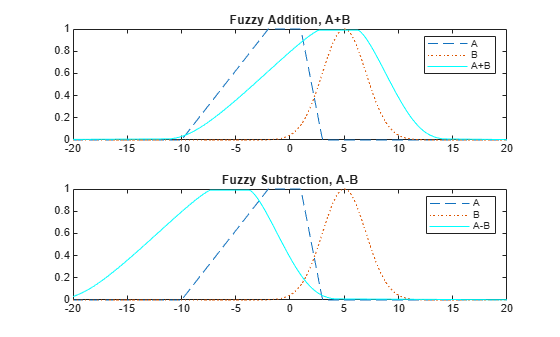fuzarith

Perform fuzzy arithmetic

Description

example

C = fuzarith(X,A,B,operator) returns the fuzzy set C, which is the result of applying the specified fuzzy operator to the fuzzy sets A and B. The operation is performed across the universe of discourse X

Examples

collapse all

Specify Gaussian and trapezoidal membership functions.

N = 501;
minX = -20;
maxX = 20;
x = linspace(minX,maxX,N);

A = trapmf(x,[-10 -2 1 3]);
B = gaussmf(x,[2 5]);

Evaluate the sum, difference, product, and quotient of A and B.

Csum = fuzarith(x,A,B,'sum');
Csub = fuzarith(x,A,B,'sub');
Cprod = fuzarith(x,A,B,'prod');
Cdiv = fuzarith(x,A,B,'div');

Plot the addition and subtraction results.

figure
subplot(2,1,1)
plot(x,A,'--',x,B,':',x,Csum,'c')
legend('A','B','A+B')
subplot(2,1,2)
plot(x,A,'--',x,B,':',x,Csub,'c')
title('Fuzzy Subtraction, A-B')
legend('A','B','A-B')Plot the multiplication and division results.

figure
subplot(2,1,1)
plot(x,A,'--',x,B,':',x,Cprod,'c')
title('Fuzzy Multiplication, A*B')
legend('A','B','A*B')
subplot(2,1,2)
plot(x,A,'--',x,B,':',x,Cdiv,'c')
title('Fuzzy Division, A/B')
legend('A','B','A/B')Input Arguments

collapse all

Universe of discourse, specified as a vector.

Input fuzzy set, specified as a vector with the same length as X. Each element of A is the value of the fuzzy set for the corresponding value of X.

A must be a convex fuzzy set. For more information, see Algorithms.

Input fuzzy set, specified as a vector with the same length as X. Each element of B is the value of the fuzzy set for the corresponding value of X.

B must be a convex fuzzy set. For more information, see Algorithms.

Arithmetic operator, specified as one of the following:

• 'sub' — Fuzzy subtraction

• 'prod' — Fuzzy multiplication

• 'div' — Fuzzy division

Note

Fuzzy addition can generate the message "divide by zero". However, this warning does not affect the accuracy of fuzarith.

Output Arguments

collapse all

Output fuzzy set, returned as a column vector with length equal to the length of X.

Algorithms

To perform fuzzy arithmetic operations, the fuzzy operands (input fuzzy sets A and B) must be convex fuzzy sets. A fuzzy set is convex if, for each pair of points x1 and x2 in the universe of discourse X and λ∈[0,1].

$\mu \left(\lambda {x}_{1}+\left(1-\lambda \right){x}_{2}\right)\ge \mathrm{min}\left(\mu \left({x}_{1}\right),\mu \left({x}_{2}\right)\right)$

An α-cut of a fuzzy set is the region in the universe of discourse for which the fuzzy set has a specific membership value, α. For a convex fuzzy set, every α-cut defines a continuous region in the universe of discourse.

fuzarith uses the continuous regions defined by the α-cuts of fuzzy sets A and B to compute the corresponding α-cut of the output fuzzy set C. To do so, fuzarith uses interval arithmetic.

The following table shows how to compute the left and right boundaries of the output interval. Here:

• [AL AR] is the interval defined by the α-cut of fuzzy set A.

• [BL BR] is the interval defined by the α-cut of fuzzy set B.

• [CL CR] is the interval defined by the α-cut of fuzzy set C.

Interval Arithmetic OperatorDefinition

$\begin{array}{l}{C}_{L}={A}_{L}+{B}_{L}\\ {C}_{R}={A}_{R}+{B}_{R}\end{array}$

Subtraction: C = A-B

$\begin{array}{l}{C}_{L}={A}_{L}-{B}_{R}\\ {C}_{R}={A}_{R}-{B}_{L}\end{array}$

Multiplication: C = A*B

$\begin{array}{l}{C}_{L}=\mathrm{min}\left({A}_{L}\cdot {B}_{L},{A}_{L}\cdot {B}_{R},{A}_{R}\cdot {B}_{L},{A}_{R}\cdot {B}_{R}\right)\\ {C}_{R}=\mathrm{max}\left({A}_{L}\cdot {B}_{L},{A}_{L}\cdot {B}_{R},{A}_{R}\cdot {B}_{L},{A}_{R}\cdot {B}_{R}\right)\end{array}$

Division: C = A/B

$\begin{array}{l}{C}_{L}=\mathrm{min}\left(\frac{{A}_{L}}{{B}_{L}},\frac{{A}_{L}}{{B}_{R}},\frac{{A}_{R}}{{B}_{L}},\frac{{A}_{R}}{{B}_{R}}\right)\\ {C}_{R}=\mathrm{max}\left(\frac{{A}_{L}}{{B}_{L}},\frac{{A}_{L}}{{B}_{R}},\frac{{A}_{R}}{{B}_{L}},\frac{{A}_{R}}{{B}_{R}}\right)\end{array}$